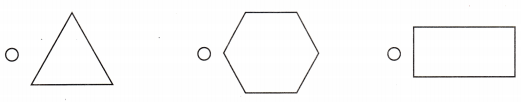# Texas Go Math Grade 2 Lesson 14.6 Answer Key Sort Two-Dimensional Shapes

Refer to our Texas Go Math Grade 2 Answer Key Pdf to score good marks in the exams. Test yourself by practicing the problems from Texas Go Math Grade 2 Lesson 14.6 Answer Key Sort Two-Dimensional Shapes.

## Texas Go Math Grade 2 Lesson 14.6 Answer Key Sort Two-Dimensional Shapes

Explore

Useto color the quadrilaterals.
Useto color the pentagons.
Use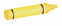1 to color the hexagons.FOR THE TEACHER • Have children use crayons to identify the shapes.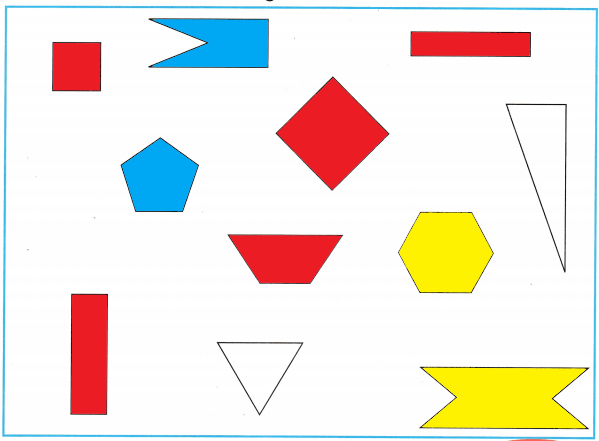Explanation:
I colored quadrilateral red, pentagons blue and the hexagons yellow.

Math Talk
Mathematical Processes

What is a sorting rule that would include all the shapes? Explain.

Model and Draw

Which shapes have fewer than 8 sides? Circle them.Explanation:
I circled the shapes that have fewer sides than 8.

Share and Show

Question 1.
Circle the shapes that have exactly 4 sides. Draw an X on the shapes that have more than 4 vertices.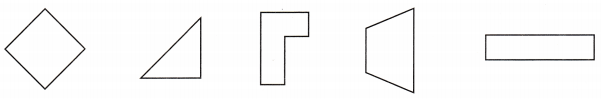Explanation:
I circled the shapes that have exactly 4 sides and marked X on the shapes that have more than 4 vertices.

Question 2.
Circle the shapes that have more than 4 sides. Draw an X on the shapes that have exactly 4 vertices.Explanation:
I circled the shapes that have more than 4 sides and drew X on the shapes that have exactly 4 vertices.

Question 3.
Circle the shapes that have exactly 6 vertices. Draw an X on the shapes that have fewer than 6 sides.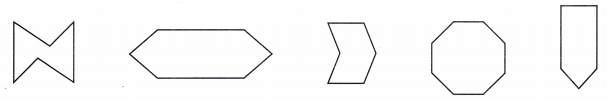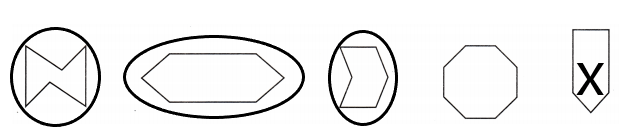Explanation:
I circled the shapes that have exactly 6 vertices and drew an X on the shapes that have fewer than 6 sides.

Problem Solving

Question 4.
Circle the shapes that have more than 8 sides. ‘ Draw an X on the shapes that have exactly 8 vertices.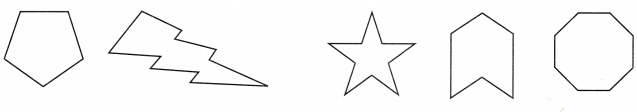Explanation:
I circled the shapes that have more than 8 sides and drew an X on the shapes that have exactly 8 vertices.

Question 5.
Multi-Step Mitch is playing a shapes game. Each side is worth a nickel. Circle the shapes that are each worth 25¢.Explanation:
Mitch is playing a shapes game. Each side is worth a nickel
Each nickel is equal to 5 cents. So, i circled the objects with 5 sides
That means i circled the shapes that are each worth 25¢.

Question 6.
H.O.T. Multi-Step Draw three shapes that match the rule. Circle them. Then draw two shapes that do not match the rule.
Shapes with fewer than 6 sides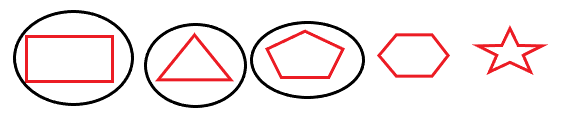Explanation:
I drew 3 shapes with fewer than 6 sides and 2 shapes with more than 6 sides.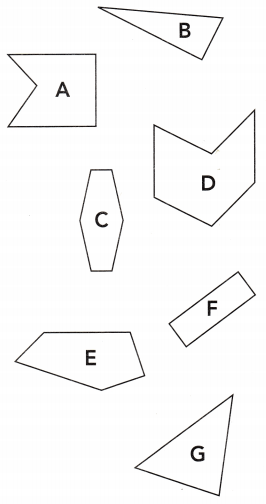Question 7.
Use Diagrams Which of these shapes has exactly 6 sides?
(A) F
(B) C
(C) A
(B) A

Explanation:
The shape C has exactly 6 sides.

Question 8.
Which shape belongs in a group of shapes that have fewer than 5 vertices?
(A) A
(B) G
(C) D
(B) G

Explanation:
The shape G has fewer than 5 vertices.

Question 9.
Use Diagrams Which of these shapes has exactly 5 vertices?
(A) D
(B) B
(C) E
(C) E

Explanation:
The shape   e has exactly 5 vertices.

Question 10.
Texas Test Prep Which shape matches this sorting rule? Shapes with more than 6 sides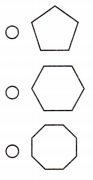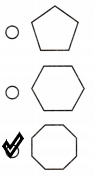Explanation:
I marked the shape that has more than 6 sides.

TAKE HOME ACTIVITY • Have your child describe some of the shapes in this lesson.

### Texas Go Math Grade 2 Lesson 14.6 Homework and Practice Answer Key

Question 1.
Circle the shapes that have fewer than 8 sides. Draw an X on the shapes that have more than 8 sides.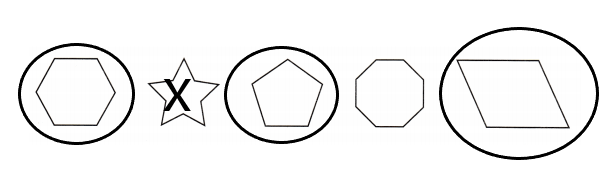Explanation:
I circled the shapes that have fewer than 8 sides and drew an X on the shapes that have more than 8 sides.

Question 2.
Circle the shapes that have exactly 3 sides. Draw an X on the shapes that have more than 3 vertices.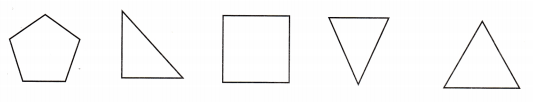Explanation:
I circled the shapes that have exactly 3 sides and drew an X on the shapes that have more than 3 vertices.

Problem Solving

Question 3.
Multi-Step Draw three shapes that match the rule. Circle them. Then draw two shapes that do not match the rule.
Shapes with fewer than 5 sides.Explanation:
I drew 3 shapes that has fewer than 5 sides and 2 shapes that has equal or more than 5 sides.

Lesson Check

Use the shapes in the box for Exercises 4-6.Question 4.
Eric sorts a group of shapes that have exactly 3 sides. How many shapes are in the group?
(A) 5
(B) 2
(C) 3
(C) 3

Explanation:
There are 3 shapes in the group that has exactly 3 sides.

Question 5.
Amber sorts a group of pentagons. How many shapes are in the group?
(A) 2
(B) 3
(C) 1
(A) 2

Explanation:
There are 2 pentagons in the group.

Question 6.
Justin sorts a group of shapes that have 6 vertices. How many shapes are in the group?
(A) 2
(B) 1
(C) 3
(B) 1

Explanation:
There is 1 shape in the group that has exactly 6 vertices.

Question 7.
Which shape matches this sorting rule? Shapes with more than 5 sides.